Examples

Chapter 10 Class 12 Vector Algebra
Serial order wise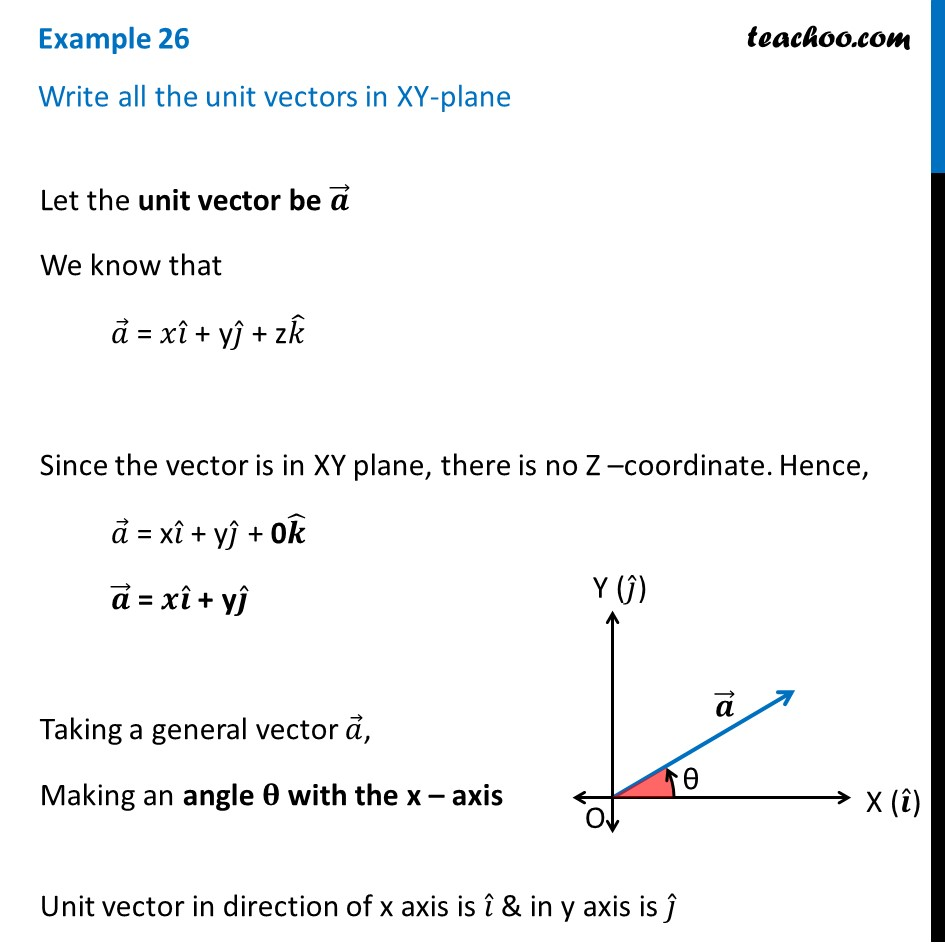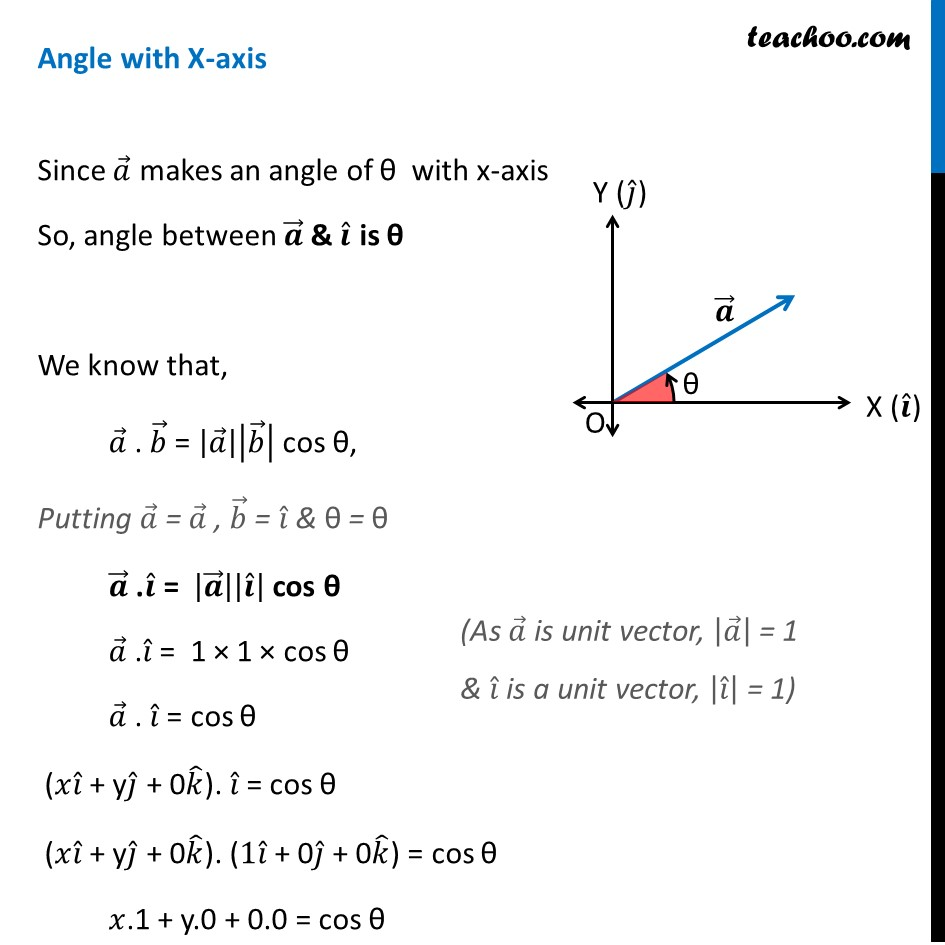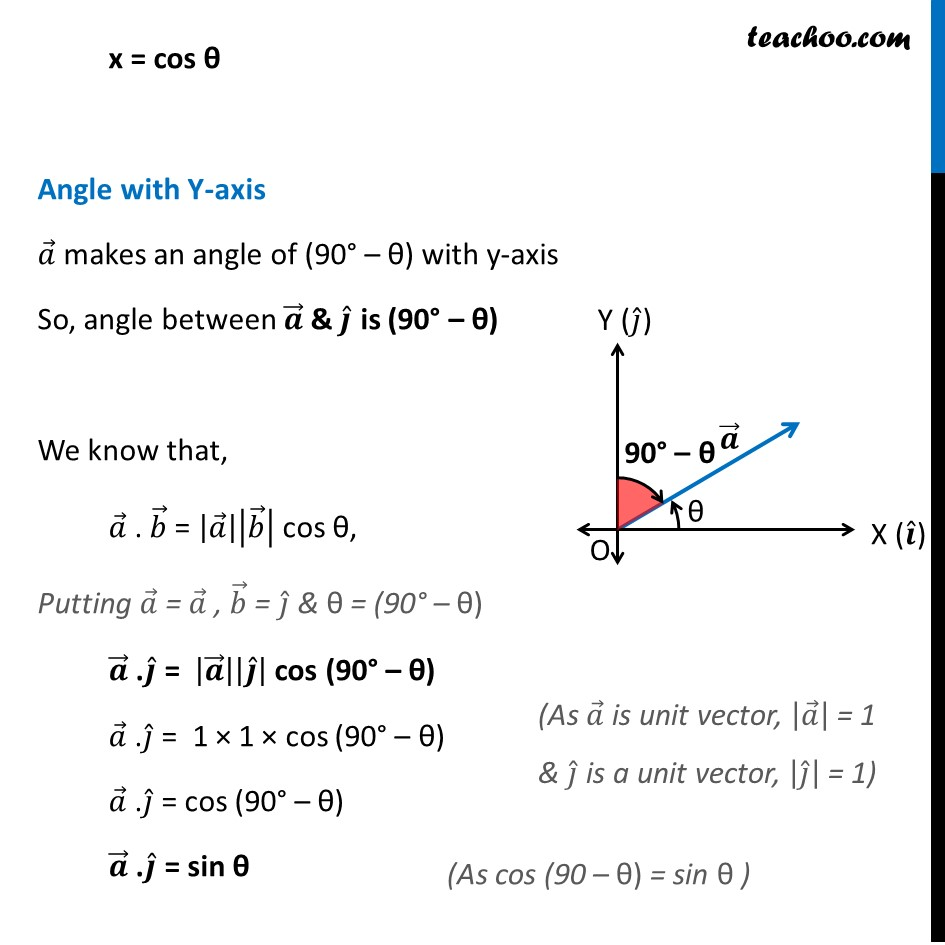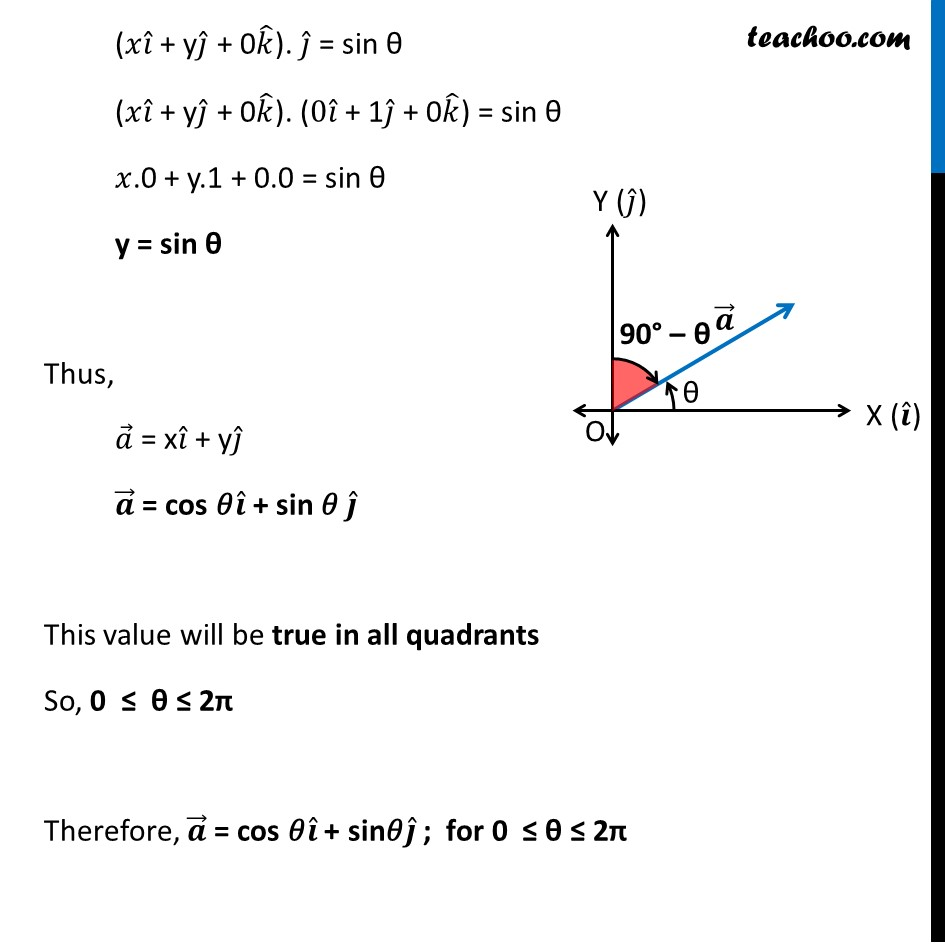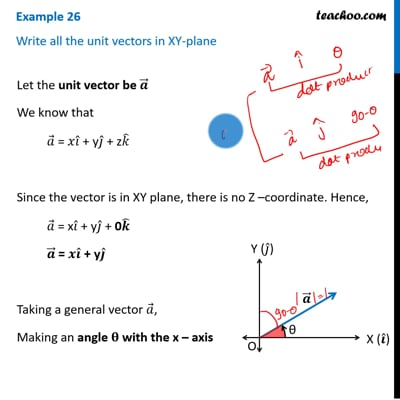This video is only available for Teachoo black users

Solve all your doubts with Teachoo Black (new monthly pack available now!)

### Transcript

= 𝑖 ̂(1 − (-4)) − j ̂ (3 − 4) + 𝑘 ̂ (−3 −1) = 𝑖 ̂(1 + 4) − j ̂ (−1) + 𝑘 ̂ (−4) = 5𝒊 ̂ + 𝒋 ̂ − 4𝒌 ̂ Magnitude of 𝑎 ⃗ × 𝑏 ⃗ = √(52+1^2+(−4)2) |𝒂 ⃗ × 𝒃 ⃗ | = √(25+1+16) = √𝟒𝟐 Area of parallelogram ABCD = |𝑎 ⃗ × 𝑏 ⃗ | = √42 Therefore, the required area is √𝟒𝟐 . Let the unit vector be 𝒂 ⃗ We know that 𝑎 ⃗ = 𝑥𝑖 ̂ + y𝑗 ̂ + z𝑘 ̂ Since the vector is in XY plane, there is no Z –coordinate. Hence, 𝑎 ⃗ = x𝑖 ̂ + y𝑗 ̂ + 0𝒌 ̂ 𝒂 ⃗ = 𝒙𝒊 ̂ + y𝒋 ̂ Taking a general vector 𝑎 ⃗, Making an angle 𝛉 with the x – axis Unit vector in direction of x axis is 𝑖 ̂ & in y axis is 𝑗 ̂ Angle with X-axis Since 𝑎 ⃗ makes an angle of θ with x-axis So, angle between 𝒂 ⃗ & 𝒊 ̂ is θ We know that, 𝑎 ⃗ . 𝑏 ⃗ = |𝑎 ⃗ ||𝑏 ⃗ | cos θ, Putting 𝑎 ⃗ = 𝑎 ⃗ , 𝑏 ⃗ = 𝑖 ̂ & θ = θ 𝒂 ⃗ .𝒊 ̂ = |𝒂 ⃗ ||𝒊 ̂ | cos θ 𝑎 ⃗ .𝑖 ̂ = 1 × 1 × cos θ 𝑎 ⃗ . 𝑖 ̂ = cos θ (𝑥𝑖 ̂ + y𝑗 ̂ + 0𝑘 ̂). 𝑖 ̂ = cos θ (𝑥𝑖 ̂ + y𝑗 ̂ + 0𝑘 ̂). (1𝑖 ̂ + 0𝑗 ̂ + 0𝑘 ̂) = cos θ 𝑥.1 + y.0 + 0.0 = cos θ (As 𝑎 ⃗ is unit vector, |𝑎 ⃗ | = 1 & 𝑖 ̂ is a unit vector, |𝑖 ̂ | = 1) x = cos θ Angle with Y-axis 𝑎 ⃗ makes an angle of (90° – θ) with y-axis So, angle between 𝒂 ⃗ & 𝒋 ̂ is (90° – θ) We know that, 𝑎 ⃗ . 𝑏 ⃗ = |𝑎 ⃗ ||𝑏 ⃗ | cos θ, Putting 𝑎 ⃗ = 𝑎 ⃗ , 𝑏 ⃗ = 𝑗 ̂ & θ = (90° – θ) 𝒂 ⃗ .𝒋 ̂ = |𝒂 ⃗ ||𝒋 ̂ | cos (90° – θ) 𝑎 ⃗ .𝑗 ̂ = 1 × 1 × cos (90° – θ) 𝑎 ⃗ .𝑗 ̂ = cos (90° – θ) 𝒂 ⃗ .𝒋 ̂ = sin θ (As 𝑎 ⃗ is unit vector, |𝑎 ⃗ | = 1 & 𝑗 ̂ is a unit vector, |𝑗 ̂ | = 1) (𝑥𝑖 ̂ + y𝑗 ̂ + 0𝑘 ̂). 𝑗 ̂ = sin θ (𝑥𝑖 ̂ + y𝑗 ̂ + 0𝑘 ̂). (0𝑖 ̂ + 1𝑗 ̂ + 0𝑘 ̂) = sin θ 𝑥.0 + y.1 + 0.0 = sin θ y = sin θ Thus, 𝑎 ⃗ = x𝑖 ̂ + y𝑗 ̂ 𝒂 ⃗ = cos 𝜃𝒊 ̂ + sin 𝜃 𝒋 ̂ This value will be true in all quadrants So, 0 ≤ θ ≤ 2π Therefore, 𝒂 ⃗ = cos 𝜃𝒊 ̂ + sin𝜃𝒋 ̂ ; for 0 ≤ θ ≤ 2π